# Comparing - math word problems

#### Number of problems found: 119

• Comparing powers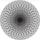How many times is number 56 larger than number 46?
• Comparing and sortingArrange in descending order this fractions: 2/7, 7/10 & 1/2
• Compare: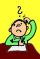What is bigger? ?
• Im>0?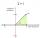Is -10i a positive number?
• Compare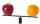Compare with characters >, <, =: 85.57 ? 80.83
• Angles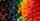Which of those angles are obtuse?
• DigitsWrite the smallest and largest 1-digit number.
• PercentsHow many percents is 900 greater than the number 750?
• CompareCompare fractions ?. Which fraction of the lower?
• Televisions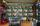Television is the fifth most expensive and tenth cheapest TV. How many different TVs are in stores?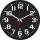Peter's badminton class starts a quarter past two PM. He came at 13:16 . Does Peter arrive on time?
• Garden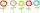The garden grows 312 roses and 389 tulips. Which flowers are more? How many?
• Snow balls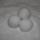Adam made 26 snowballs. Boris made less snowballs. How many snowballs Boris could do?
• Equivalent fractionsAre these two fractions equivalent -4/9 and 11/15?
• Opposite numbers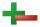Calculate opposite numbers (additive inverse) to given ones:How much and how many times is 72.1 greater than 0.00721?
• Compare IIWhich of the numbers 710, 107 is greater?
• Package Deal - what to choose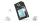The parents decided to purchase a mobile phone to their son. They choose from menu: ? Which offer is best?
• Possible lengths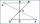Find the most possible lengths for the third side of a triangle with sides 20 and 18.
• IntegerFind the integer whose distance on the numerical axis from number 1 is two times smaller as the distance from number 6.

Do you have an interesting mathematical word problem that you can't solve it? Submit a math problem, and we can try to solve it.

We will send a solution to your e-mail address. Solved examples are also published here. Please enter the e-mail correctly and check whether you don't have a full mailbox.

Please do not submit problems from current active competitions such as Mathematical Olympiad, correspondence seminars etc...